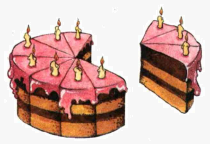What is Fraction

Related calculator: Fractions CalculatorSo, what is fraction?

Suppose you divided a cake into 10 pieces and eat 3 pieces. How much of cake do you eat?

We write it as $\frac{{3}}{{10}}$.

3 is a number of pieces you eat and 10 is total number of pieces.

This is new situation because 3 is not divisible by 10 $\left(3<10\right)$.

A fraction is a way to represent division of integer into parts: $\frac{{n}}{{m}}$ is a fraction where both ${n}$ and ${m}$ are integers.

${n}$ is called numerator, ${m}$ is called denominator: $\frac{{\overbrace{{\color{green}{{{n}}}}}^{{{\color{green}{{\text{numerator}}}}}}}}{{\underbrace{\color{purple}{{{m}}}}_{{{\color{purple}{{\text{denominator}}}}}}}}$.

So, fractions arise when one number is not divisible by another: since 19 is not divisible by 3, but we want to divide them, we write $\frac{{19}}{{3}}$.

When integer is divisible by another integer we can write it as fraction, but it is more natural to write it as integer: $\frac{{8}}{{4}}={2}$.

Note, that every integer ${b}$ can be represented as a fraction: $\color{purple}{{{b}=\frac{{b}}{{1}}}}$.

For example, ${5}=\frac{{5}}{{1}}$.

In case when numerator and denominators are equal we obtain one as result: $\color{red}{{\frac{{m}}{{m}}={1}}}$.

For example, $\frac{{10}}{{10}}={1}$.

Really, we divide a cake into 10 pieces and eat all 10 pieces - so we eat all cake.